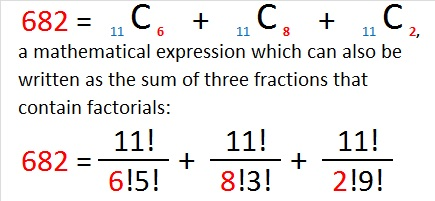# 682 Deserves a Lot of Exclamation Points!!!

682 is the sum of the four prime numbers from 163 to 179. 682 is also the sum of the ten prime numbers from 47 to 89.

6 – 8 + 2 = 0 so 682 is divisible by 11.

Stetson.edu shared another amazing relationship between the number 682 and the number 11:Besides the obvious inclusion of the digits 6-8-2, notice in the factorial expression that 11 is in each numerator and that 11 is also the sum of the numbers in each denominator.Print the puzzles or type the solution on this excel file: 12 Factors 2015-11-16

—————————————————————————————————

• 682 is a composite number.
• Prime factorization: 682 = 2 x 11 x 31
• The exponents in the prime factorization are 1, 1, and 1. Adding one to each and multiplying we get (1 + 1)(1 + 1)(1 + 1) = 2 x 2 x 2 = 8. Therefore 682 has exactly 8 factors.
• Factors of 682: 1, 2, 11, 22, 31, 62, 341, 682
• Factor pairs: 682 = 1 x 682, 2 x 341, 11 x 62, or 22 x 31
• 682 has no square factors that allow its square root to be simplified. √682 ≈ 26.1151297.—————————————————————————————————